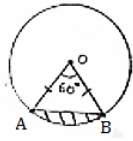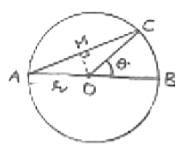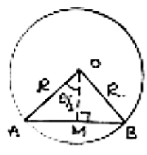# RD Sharma Class 10 Ex 15.4 Solutions Chapter 15 Areas Related To Circles

In this chapter, we provide RD Sharma Class 10 Ex 15.4 Solutions Chapter 15 Areas Related To Circles for English medium students, Which will very helpful for every student in their exams. Students can download the latest RD Sharma Class 10 Ex 15.4 Solutions Chapter 15 Areas Related To Circles pdf, Now you will get step by step solution to each question.

# Chapter 15: Areas Related To Circles Exercise – 15.4

### Question: 1

AB is a chord of a circle with centre O and radius 4 cm. AB is length 4 cm and divides circle into two segments. Find the area of minor segment

### Solution:Radius of circle r = 4cm = OA = OB

Length of chord AB = 4cm

OAB is equilateral triangle ∠AOB = 60° → θ

Angle subtended at centre θ = 60°

Area of segment (shaded region) = (Area of sector) – (Area of ∆ AOB)

### Question: 2

A chord of circle of radius 14 cm makes a light angle at the centre. Find the areas of minor and major segments of the circle.

### Solution:θ = 90°

= OA = OB

Area of minor segment (ANB) = (Area of ANB sector) – (Area of MOB)

Area of major segment (other than shaded) = Area of circle – Area of segment ANB

= πr2 – 56

= 22/7 × 14 × 14 – 56

= 616 – 56

= 560 cm2.

### Question: 3

A chord 10 cm long is drawn in a circle whose radius is 5√2 cm. Find the area of both segments

### Solution:

Given radius = r = 5√2 cm= OA = OB

Length of chord AB = 10 cm

In ∠OAB,

OA = OB = 5√2 cm AB = 10 cm

OA2 + OB2 = (5√2)2 + (5√2)2

= 50 + 50 = 100 = (AB)2

Pythagoras theorem is satisfied OAB is light triangle

θ = Angle subtended by chord = ∠AOB = 90°

Area of segment (minor) = Shaded region = Area of sector – Area of ∆OAB

Area of major segment = (Area of circle) – (Area of minor segment)

### Question: 4

A chord AB of circle, of radius 14cm makes an angle of 60° at the centre. Find the area of minor segment of circle.

### Solution:

Given radius (r) = 14 cm = OA = OBθ = angle at centre = 60°

In AAOB, ∠A = ∠B [angles opposite to equal sides OA and OB] = x

By angle sum property ∠A + ∠B + ∠O = 180°

x + x + 60° = 180°

⟹ 2x = 120°

⟹ x = 60°

All angles are 60°, OAB is equilateral OA = OB = AB

Area of segment = Area of sector – Area. ∆le OAB

### Question: 5

AB is the diameter of a circle, centre O. C is a point on the circumference such that ∠COB = θ. The area of the minor segment cutoff by AC is equal to twice the area of sector BOC. Prove that

### Solution:

Given AB is diameter of circle with centre O∠COB = θ

Area of segment cut off, by AC = (Area of sector) – (Area of ∆AOC)

∠AOC = 180 – θ [∠AOC and ∠BOC form linear pair]

Area of segment by AC = 2 (Area of sector BDC)

Area of segment by AC = 2 (Area of sector BDC)

### Question: 6

A chord of a circle subtends an angle O at the centre of circle. The area of the minor segment cut off by the chord is one eighth of the area of circle. Prove that 8 sin θ/2. cos θ/2 + π = π θ/45.

### Solution:

Let radius of circle = rArea of circle = πr2

AB is a chord, OA, OB are joined drop OM ⊥ AR This OM bisects AB as well as ∠AOB.

∠AOM = ∠MOB = 1/2(0) = θ/2 AB = 2AM

In ∆AOM, ∠AMO = 90°

Area of segment cut off by AB = (Area of sector) – (Area of triangles)

Area of segment = 1/2 (Area of circle)

All Chapter RD Sharma Solutions For Class10 Maths

I think you got complete solutions for this chapter. If You have any queries regarding this chapter, please comment on the below section our subject teacher will answer you. We tried our best to give complete solutions so you got good